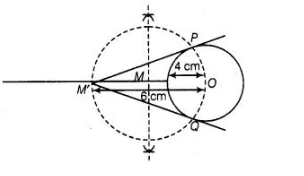# Construct a tangent to a circle of radius 4 cm

Question:

Construct a tangent to a circle of radius 4 cm from a point which is at a distance of 6 cm from its centre.

Solution:

Given, a point M’ is at a distance of 6 cm from the centre of a circle of radius 4 cm.Steps of construction

1. Draw a circle of radius $4 \mathrm{~cm}$. Let centre of this circle is $\mathrm{O}$.

2. Join OM' and bisect it. Let $M$ be mid-point of OM'.

3. Taking $M$ as centre and $M O$ as radius draw a circle to intersect circle $(0,4)$ at two points, $P$ and $Q$.

4. Join $P M^{\prime}$ and $Q M^{\prime} . P M^{\prime}$ and $Q M^{\prime}$ are the required tangents from $M^{\prime}$ to circle $C(0,4)$.